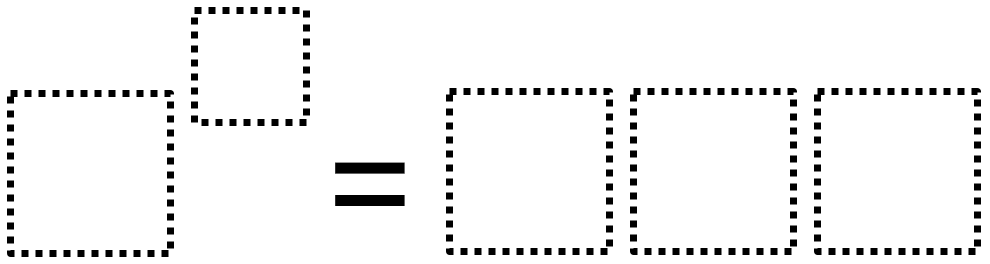# Exponent (Maximum Value)

Directions: Using the digits 1 to 9, at most one time each, fill in the boxes to make a result that has the greatest value possible.### Hint

How does changing the base affect the value?
How does changing the exponent affect the value?

Initially, some students may think that the answer is 8^9. This breaks the structure of the problem, as the result has to be 3-digits.

The largest possible 3-digit result is 729 from 3^6 = 729.

Source: Robert Kaplinsky

## Square Root Expression 2

Directions: Using the digits 0 to 9 at most one time each, place a digit …

1.•